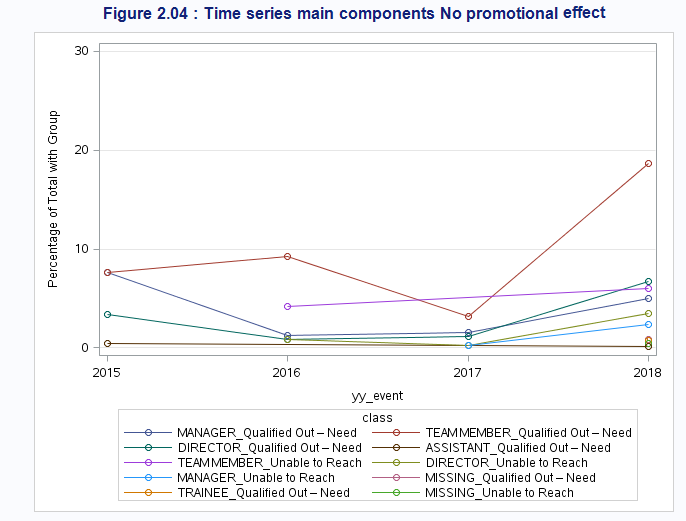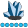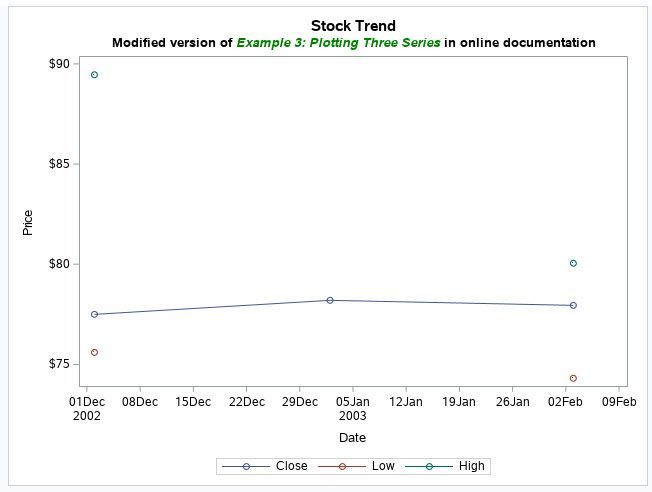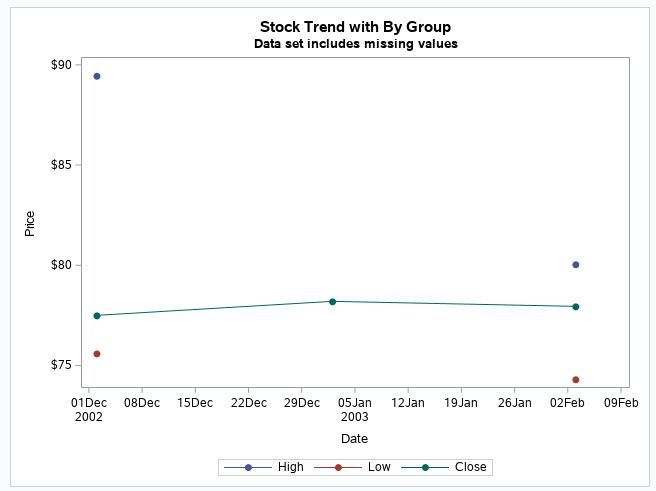## Proc Sgplot : Break option Not working

Hi All

With the following code below I get the below series graph.

The Issue I'm facing is that for the Team_Member_Unable_to_reach category, between the years 2016 and 2018, the line

does not break as It should be, as for that specific category there are no lines in the dataset (the combination is missing )

Should not the break option allow for the line to break into two dots? Any idea on what could be the issue?

Thanks

Bests``````title 'Figure 2.04 : Time series main components No promotional effect ';

proc sgplot data=FreqOut (where=(outcome_rev like '%No_sales_gen_effec%'));
series x=yy_event y=Percent /markers group=class grouporder=data break nomissinggroup ;
xaxis type=discrete;
yaxis grid values=(0 to 30 by 10) label="Percentage of Total with Group";
run;``````

1 ACCEPTED SOLUTION

Accepted SolutionsSuzanneDorinski
Lapis Lazuli | Level 10

## Re: Proc Sgplot : Break option Not working

I think you need observations with missing values for the BREAK option to have an effect.

The example below produces three graphics.  The BREAK option has an effect in two of the three graphics.  The graphic where BREAK doesn't have an effect is a data set with no missing values.

``````data ibm;
set sashelp.stocks(where=("02dec2002"d <= date <= "03feb2003"d and stock='IBM'));
if date="02jan2003"d then
do;
high=.;
low=.;
end;
run;

out=transposed_ibm(rename=(_name_=type col1=price));
by stock date notsorted;
run;

proc sgplot data=ibm;
title "Stock Trend";
title2 'Modified version of '
italic color=green 'Example 3: Plotting Three Series'
font='Albany AMT' bold color=black ' in online documentation';

series x=date y=close / markers break;
series x=date y=low / markers break;
series x=date y=high / markers break;

yaxis label='Price';
run;

proc sgplot data=transposed_ibm(where=(type in ('Close','Low','High')));
title "Stock Trend with By Group";
title2 'Data set includes missing values';

series x=date y=price / markers
markerattrs=(symbol=circlefilled)
group=type
break
nomissinggroup;

yaxis label='Price';
keylegend / title='';
run;

data ibm_no_missing;
set transposed_ibm;
if price = . then delete;
run;

proc sgplot data=ibm_no_missing(where=(type in ('Close','Low','High')));
title "Stock Trend with By Group";
title2 "Missing values removed from data set";

series x=date y=price / markers
markerattrs=(symbol=circlefilled)
group=type
break
nomissinggroup;

yaxis label='Price';
keylegend / title='';
run;``````3 series plots, two series with missing values, BREAK has an effectSeries plot with grouped data, two groups have observations with missing values, BREAK has an effectSeries plot with grouped data, missing values deleted from data set, BREAK has no effect

5 REPLIES 5

## Re: Proc Sgplot : Break option Not working

If I am understanding your question, here is an example that demonstrates that the BREAK option is working as expected.  Perhaps your data has a different form? You can modify the example to illustrate the situation that you are seeing.

``````data Have;
input Class \$ Year Y;
datalines;
A 2015 1
A 2016 2
A 2017 1
A 2018 3
B 2015 .
B 2016 1
B 2017 .
B 2018 2
;

proc sgplot data=Have;
series x=Year y=Y /markers group=class grouporder=data break nomissinggroup ;
xaxis type=discrete;
yaxis grid ;
run;``````

## Re: Proc Sgplot : Break option Not working

Hi Richard

In my dataset there are no observations for the missing combination category/period. Using your example, It is like:

``````data Have;
input Class \$ Year Y;
datalines;A 2015 1
A 2016 2
A 2017 1
A 2018 3

B 2016 1

B 2018 2;
``````SuzanneDorinski
Lapis Lazuli | Level 10

## Re: Proc Sgplot : Break option Not working

I think you need observations with missing values for the BREAK option to have an effect.

The example below produces three graphics.  The BREAK option has an effect in two of the three graphics.  The graphic where BREAK doesn't have an effect is a data set with no missing values.

``````data ibm;
set sashelp.stocks(where=("02dec2002"d <= date <= "03feb2003"d and stock='IBM'));
if date="02jan2003"d then
do;
high=.;
low=.;
end;
run;

out=transposed_ibm(rename=(_name_=type col1=price));
by stock date notsorted;
run;

proc sgplot data=ibm;
title "Stock Trend";
title2 'Modified version of '
italic color=green 'Example 3: Plotting Three Series'
font='Albany AMT' bold color=black ' in online documentation';

series x=date y=close / markers break;
series x=date y=low / markers break;
series x=date y=high / markers break;

yaxis label='Price';
run;

proc sgplot data=transposed_ibm(where=(type in ('Close','Low','High')));
title "Stock Trend with By Group";
title2 'Data set includes missing values';

series x=date y=price / markers
markerattrs=(symbol=circlefilled)
group=type
break
nomissinggroup;

yaxis label='Price';
keylegend / title='';
run;

data ibm_no_missing;
set transposed_ibm;
if price = . then delete;
run;

proc sgplot data=ibm_no_missing(where=(type in ('Close','Low','High')));
title "Stock Trend with By Group";
title2 "Missing values removed from data set";

series x=date y=price / markers
markerattrs=(symbol=circlefilled)
group=type
break
nomissinggroup;

yaxis label='Price';
keylegend / title='';
run;``````3 series plots, two series with missing values, BREAK has an effectSeries plot with grouped data, two groups have observations with missing values, BREAK has an effectSeries plot with grouped data, missing values deleted from data set, BREAK has no effect

## Re: Proc Sgplot : Break option Not working

Thank you Suzanne. So the Break option does not work with actual missing observations.

As the dataset it is created with a Proc Freq, I understand I should find a way to create missing observation for the actual missing combinations of Categories * PeriodPGStats
Opal | Level 21

## Re: Proc Sgplot : Break option Not working

Add missing values with something like:

``````data freqOutPlus;
retain lastEvent;
set freqOut(rename=(percent=newPercent yy_event=newEvent));
by class;
if not first.class then do;
do yy_event = lastEvent+1 to newEvent - 1;
output;
end;
yy_event = newEvent;
percent = newPercent;
output;
lastEvent = newEvent;
drop newPercent newEvent lastEvent;
run;

proc sgplot data=FreqOutPlus....``````

(untested)

PG
Discussion stats
• 5 replies
• 4498 views
• 4 likes
• 4 in conversation